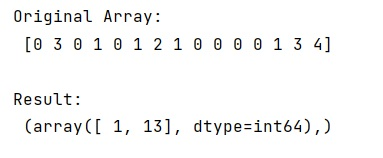# Get the first index of an elements in a NumPy array

In this tutorial, we will learn how to get the first index of an elements in a NumPy array? By Pranit Sharma Last updated : May 23, 2023

Suppose we are given a NumPy array with some values and we are given the specified item to search for.

## Getting the first index of an elements in a NumPy array

To Get the first index of an elements in a NumPy array, you can use numpy.where() by passing arr == item, where arr is NumPy array and item is the value whose index to be found.

The result of this expression would be a tuple with first all the row indices, then all the column indices.

Let us understand with the help of an example,

## Python program to get the first index of an elements in a NumPy array

```# Import numpy
import numpy as np

# Creating a numpy array
arr = np.array([0, 3, 0, 1, 0, 1, 2, 1, 0, 0, 0, 0, 1, 3, 4])

# Specifying an item to search
item = 3

# Display Original Array
print("Original Array:\n",arr,"\n")

# Using numpy.where
index = np.where(arr == item)

# Display Result
print('Result:\n',index)
```

### Output

```Original Array:
[0 3 0 1 0 1 2 1 0 0 0 0 1 3 4]

Result:
(array([ 1, 13]),)
```

### Output (Screenshot)